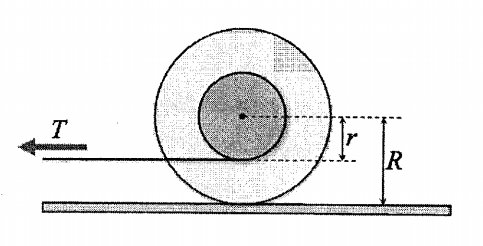# Angular acceleration of a yo-yo pulled with constant force

• ShilpaM

## Homework Statement

A yo-yo is pulled with a constant tension T. The string is horizontal and parallel to the table and unwinding from the bottom of the spool, as shown. The yo-yo's outer radius is R and the spool radius is r. The mass of the yo-yo is m and the moment of inertia of the yo-yo around the axis through its centre of mass is I. What is the angular acceleration of the yo-yo, assuming it rolls without slipping?2. Homework Equations

α = τnet / I
τ = Fsinθr

## The Attempt at a Solution

I think the spool rotates in the same direction as T, but the rest of the yo-yo has to rotate in the opposite direction in order to roll without slipping. T applies a negative torque, since it causes rotation in the CW direction, so τT = -Tr
This is where I'm stuck. Is the inertia of the yo-yo just I or is it I + mR2? And I'm not sure if it's only tension applying a torque to the yo-yo, or if the floor is exerting a backward force on the yo-yo to make it roll, and if so, is the force proportionate to the normal force. Can anyone please help me figure this one out?